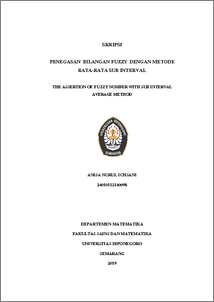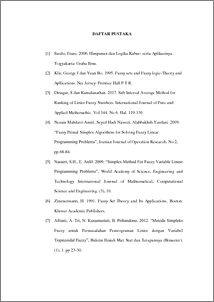# THE ASSERTION OF FUZZY NUMBER WITH SUB INTERVAL AVERAGE METHOD

Ichsani, Anisa Nurul (2019) THE ASSERTION OF FUZZY NUMBER WITH SUB INTERVAL AVERAGE METHOD. Undergraduate thesis, UNDIP.Preview
PDF
88KbPDF
Restricted to Repository staff only

418KbPreview
PDF
37Kb

## Abstract

The average sub-interval method is a method for determining the ranking value of a fuzzy set by looking at the number of intervals at all points of the sub-interval. Each sub interval has an upper and lower limit. The average of all these intervals is a ranking function. The ranking function is generally formed by calculating the mean of all average amounts between each sub-interval. This method is a simple calculation and can rank various types of fuzzy numbers. Keywords: Sub Interval Fuzzy, Number Ranking Fuzzy, Polygon Fuzzy Numbers

Item Type: Thesis (Undergraduate) Q Science > QA Mathematics Faculty of Science and Mathematics > Department of Mathematics 84240 INVALID USER 13 Jun 2022 08:21 13 Jun 2022 08:21

Repository Staff Only: item control page